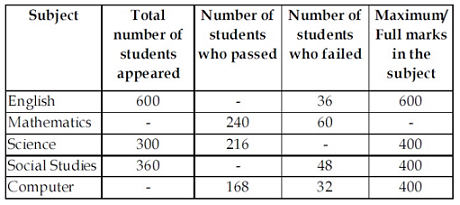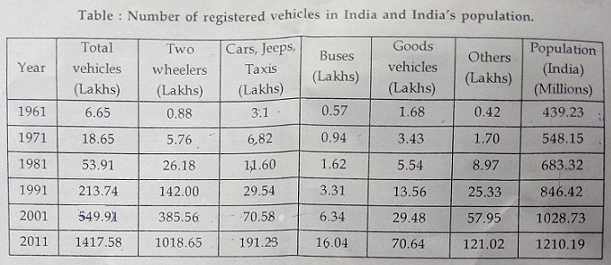1. Study the table given below carefully. It shows the number of students appeared, passed and failed in five subjects. The full marks in each subject is also given.Some of the cells have missing data. You might need to determine some of the missing data to answer the question below.Which of the following could be the full marks in Mathematics if the pass marks in Mathematics was 35% and the person who just passed scored 70?a. 400 b. 500 c. 600 d. 200

 2. Study the table given below carefully. It shows the number of students appeared, passed and failed in five subjects. The full marks in each subject is also given.Some of the cells have missing data. You might need to determine some of the missing data to answer the question below.What is the maximum mark that a student can score in all the five subjects together?a. 500 b. 1000 c. 1500 d. 2000

 3. Study the table given below carefully. It shows the number of students appeared, passed and failed in five subjects. The full marks in each subject is also given.Some of the cells have missing data. You might need to determine some of the missing data to answer the question below.What is the difference between the number of failed students in Science and the number of passed Social Studies?a. 228 b. 238 c. 312 d. 218

 4. Study the table given below carefully. It shows the number of students appeared, passed and failed in five subjects. The full marks in each subject is also given.Some of the cells have missing data. You might need to determine some of the missing data to answer the question below.What is the approximate difference in percentage between the pass % in Social Studies and the pass % in Mathematics?a. 2.5 % b. 6.5 % c. 16.5 % d. 26.5 %

 5. Study the table given below carefully. It shows the number of students appeared, passed and failed in five subjects. The full marks in each subject is also given.Some of the cells have missing data. You might need to determine some of the missing data to answer the question below.In which subject, was the failure percent the least?a. English b. Mathematics c. Science d. Social Studies

 6. Answer the following question based on the data given in the table below.The maximum decadal growth in population of India is registered in the period:a. 1981 - 1991 b. 1961 - 1971 c. 1991 - 2001 d. 2001 - 2011

 7. Answer the question based on the data given in the table below.What was the per capita ownership of two wheelers in India in the year 2011?a. ~0.068% b. ~0.084% c. ~0.0084% d. None of the above
 8. Answer the question based on the data given in the table below.What was the average decadal growth in the number of cars during 1961 – 2011?a. ~157% b. ~131% c. ~68% d. ~217%
 9. Answer the question based on the data given in the table below.In which year the decadal growth (%) in number of cars surpassed that of the two wheelers?a. 2011 b. 1991 c. 2001 d. 1981
 10. Answer the question based on the data given in the table below.In the year 2001, out of total number of vehicles, the number of passenger vehicles (4 wheelers) accounted for:a. ~43% b. ~14% c. ~24% d. ~31%